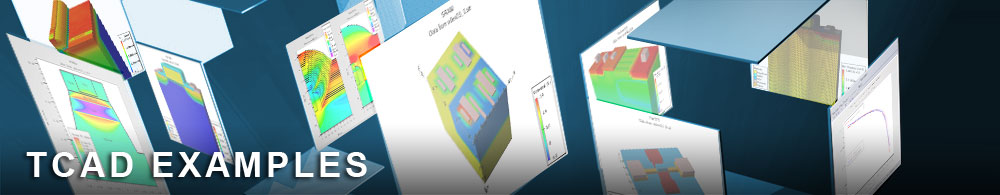Body Effect Extraction

## mos1ex12.in : Body Effect Extraction

Requires: SSuprem 4/S-Pisces
Minimum Versions: Athena 5.22.3.R, Atlas 5.28.1.R

This is a basic MOS Athena to Atlas interface example simulating two Id/Vgs curves at different substrate biases and extracting the body effect (gamma) parameter. This example demonstrates:

• Process simulation of a MOS transistor in Athena
• Process parameter extraction (eg. oxide thicknesses)
• Autointerface between Athena and Atlas
• Simple Id/Vgs curve generation with Vbs=0.0V
• Ramp of drain voltage
• Simple Id/Vgs curve generation with Vbs=1.0V
• Parameter extraction for body effect

The process simulation, process parameter extraction and electrode definition for this example are exactly as described in the first example in this section.

In Atlas the whole example is very similar in syntax to the DIBL parameter extraction example described previously in this section. The difference is that different substrate biases are used instead of different drain biases.

Two threshold voltages are measured using the extract syntax described in the DIBL extraction example. The body effect parameter is derived from the threshold voltages using the standard formula assuming 0.6V for phi.

To load and run this example, select the Load button in DeckBuild > Examples. This will copy the input file and any support files to your current working directory. Select the Run button in DeckBuild to execute the example.